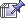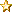August 08, 2022, 06:27:59 am
 News: ARE Search For Atlantis 2007 Results http://mysterious-america.net/bermudatriangle0.html# Hipparchus' Celestial Globe

 Pages: 1 2    Go DownAuthor Topic: Hipparchus' Celestial Globe  (Read 2727 times)
Bianca
Superhero MemberPosts: 41646« Reply #30 on: December 08, 2007, 10:08:54 am »

A.2.4. Chi-square Analysis

The best estimate for the date of the observations that were used to construct the constellations on the Farnese Atlas is that year for which the observed positions from Tables 3 and 5 most closely match the real sky. The optimal statistic for all such analyses22 is always the chi-square statistic, χ2.

The χ2 statistic is simply the summation over the data points of the squares of the deviations between the observations and the model in units of the standard deviation for the measurement. Symbolically, this is χ2 = Σ{[(Oi  Mi)/σi]2}, where the summation is over all data items, the subscript i itemizes the datum, O is the observed value, M is the model value, and σ is the measurement uncertainty. For example, for the ﬁrst datum in Table 3, for an epoch of 125 B.C., the observed right ascension of the star γ Ari is 0.5°, the model value is 0° corresponding to the vernal equinox colure, and the typical scatter near the equator implies σ = 3.5°; so the χ2 contribution for this datum is 0.02. For the ﬁrst star on the equator (item #13 in Table 1), the observed declination of σ Ari in 125 B.C. is 4.7°, the model value is 0° which corresponds to the equator, and the typical scatter is σ = 3.5°; so the χ2 contribution for this datum is 1.8. For item #56 in Table 5, the observed ecliptic longitude of α Tau in 125 B.C. is 47.6° on the Farnese Atlas, the model value is 40.2°, and the uncertainty near the equator is 5°; so the χ2 contribution for this datum is 2.2. For a given date, the χ2 contributions can be summed over all data points to produce the χ2 value.

The χ2 value is smallest for the best model. In this case, the best model would be ideally that the Farnese Atlas represents the true positions of the identiﬁed stars for some particular date. By varying the date, the χ2 will vary also, with the date of the minimum χ2 being the best estimate date. The 68% probability error bar (i.e., the one-sigma uncertainty region) is that range of dates for which the χ2 is within 1.0 of the minimum value. With this, we have a standard technique for determining the best date for the Atlas as well as quantitatively deriving the real error bar in that date.

In Tables 3 and 5, I tabulate a total of 70 data points taken from the Farnese Atlas, and these constitute the observations. The model consists of the modern positions for these same stars or target circles as calculated by precession for various years. The χ2 contributions are simply the differences between the observed and model values (divided by the measurement uncertainty) squared. The uncertainties are 3.5° in position for Table 3 and 5° in ecliptic longitude for Table 5 (both with corrections for the latitude as needed). These are summed to give the χ2 value. I have calculated the χ2 for all dates from 400 B.C. to A.D. 200 at ﬁ ve-year intervals.

The date of minimum χ2 is 125 B.C. The χ2 at minimum is 66.3.23 The χ2 rises to

67.3 for the years 70 B.C. and 180 B.C., so this is the one-sigma range for the date (i.e., there is a 68% probability that the real date is between 180 and 70 B.C.). The two-sigma range (i.e., the 95% conﬁ dence region) is that for which the χ2 is lower than 66.3 + 22 = 70.3 and is between 245 and 10 B.C. The three-sigma range (i.e., the 99.7% conﬁdence region) is that for which the χ2 is lower than 66.3 + 32 = 75.3 and is between 305 B.C. and A.D. 50. The total size for the one-, two-, and three-sigma intervals is 110, 235, and 355 years respectively. It is encouraging and expected that these interval sizes are nearly in the proportions 1:2:3. The standard way to present such results is to quote the year of minimum χ2 as the best estimate of the date and to give the uncertainty as half the size of the one-sigma region. Thus, I conclude that the constellations on the Farnese Atlas were based on observations made in the year 125 ± 55 B.C.

It is appropriate here to note that this derived date is a very conﬁ dent result. The techniques in the Appendices are all standard, straight forward and deﬁ nitive. The deviations from the simple model are all Gaussian with zero outliers. The selection of various subsamples of the data still yields the same date (to within the quoted error bars), so the result is not simply due to some number of localized errors of any type. I cannot think of any astronomical effect or error nor of any historical vagary or error that would artiﬁcially produce the derived date.

In all, to within the quoted error bars, the derived date of 125 B.C. is of high conﬁdence.
 Report SpamLogged

Your mind understands what you have been taught; your heart what is true.
 Pages: 1 2    Go Up